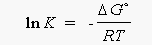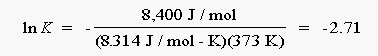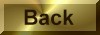Practice Problem 11

Use values ofHo andSo for the following reaction at 25°C to estimate the equilibrium constant for this reaction at the temperature of boiling water (100°C), ice(0°C), a dry ice-acetone bath (-78°C), and liquid nitrogen (-196°C):

2 NO2(g)N2O4(g)

Solution

Using a standard-state enthalpy of formation and absolute entropy data table, we find the following information:

CompoundHfo(kJ/mol)         S°(J/mol-K)

NO2(g)                 33.18            240.06

N2O4 (g)                9.16           304.29

According to these data, the reaction is favored by enthalpy:Ho = (1 mol N204 x 9.16 kJ/mol) - (2 mol  NO2 x 33.18 kJ/mol)

= -57.20 kJ

But it is not favored by entropy:So = (1 mol N204 x 304.29 J/mol-K) - (2 mol  NO2 x 240.06 J/mol-K)

= -175.83 J/K

If we assume that these values ofHo andSo are still valid at 100°C, the value ofGo at this temperature is 8400 J:Go373 =Ho298 - TSo298

= -57,200 J - (373 K)(-175.83 J/K) = 8400 J

Repeating this calculation at the other temperatures gives the following results:

100°C:Go = 8.4 kJ

0°C:Go = -9.2 kJ

-78°C:Go = -22.9kJ

-196°C:Go = -43.7 kJ

We now write the equation for the relationship betweenGo and the equilibrium constant for the reaction:Go = - RT ln K

and solve for the natural log of the equilibrium constant:Let's start by calculating the value of ln Kp when the reaction is at 100°C:The equilibrium constant at this temperature is therefore 0.067:

K = e-2.71 = 0.067

Repeating this calculation at the other temperatures gives the following results:

100°C:        Kp = 0.067

0°C:           Kp = 58

-78°C:        Kp = 1.4 x 106

-196°C:       Kp   = 3.8 x 1029

At 100°C, the unfavorable entropy term is realtively important, and the equilibrium lies on the side of NO2.  As the reaction is cooled, the entropy term becomes less important, and the equilibrium shifts toward N2O4.   At the temperature of liquid nitrogen all of the NO2 condenses to form N2O4.